# 复杂网络特征与networkx实现------（二）

## 图的类型

• Graph类是无向图的基类，无向图能有自己的属性或参数，不包含重边，允许有回路，节点可以是任何hash的python对象，节点和边可以保存key/value属性对。该类的构造函数为Graph(data=None，**attr)，其中data可以是边列表，或任意一个Networkx的图对象，默认为none；attr是关键字参数，例如key=value对形式的属性。
import networkx as nx      #导入NetworkX包，为了少打几个字母，将其重命名为nx
G = nx.Graph()              #建立一个空的无向图G
• MultiGraph是可以有重边的无向图，其它和Graph类似。其构造函数MultiGraph（data=None, *attr）。

• DiGraph是有向图的基类，有向图可以有数自己的属性或参数，不包含重边，允许有回路；节点可以是任何hash的python对象，边和节点可含key/value属性对。该类的构造函数DiGraph(data=None,**attr)，其中data可以是边列表，或任意一个Networkx的图对象，默认为none；attr是关键字参数，例如key=value对形式的属性。

• MultiDiGraph是可以有重边的有向图，其它和DiGraph类似。其构造函数MultiDiGraph（data=None, *attr）。

## 基本概念

• 距离
最短距离：两节点${v}_{i}$$v_i$和节点${v}_{j}$$v_j$经历边数最少的一条简单路径
直径：任意两个节点距离的最大值$D=ma{x}_{i,j}{d}_{\left(}ij\right)$$D= max_{i,j}{d_(ij)}$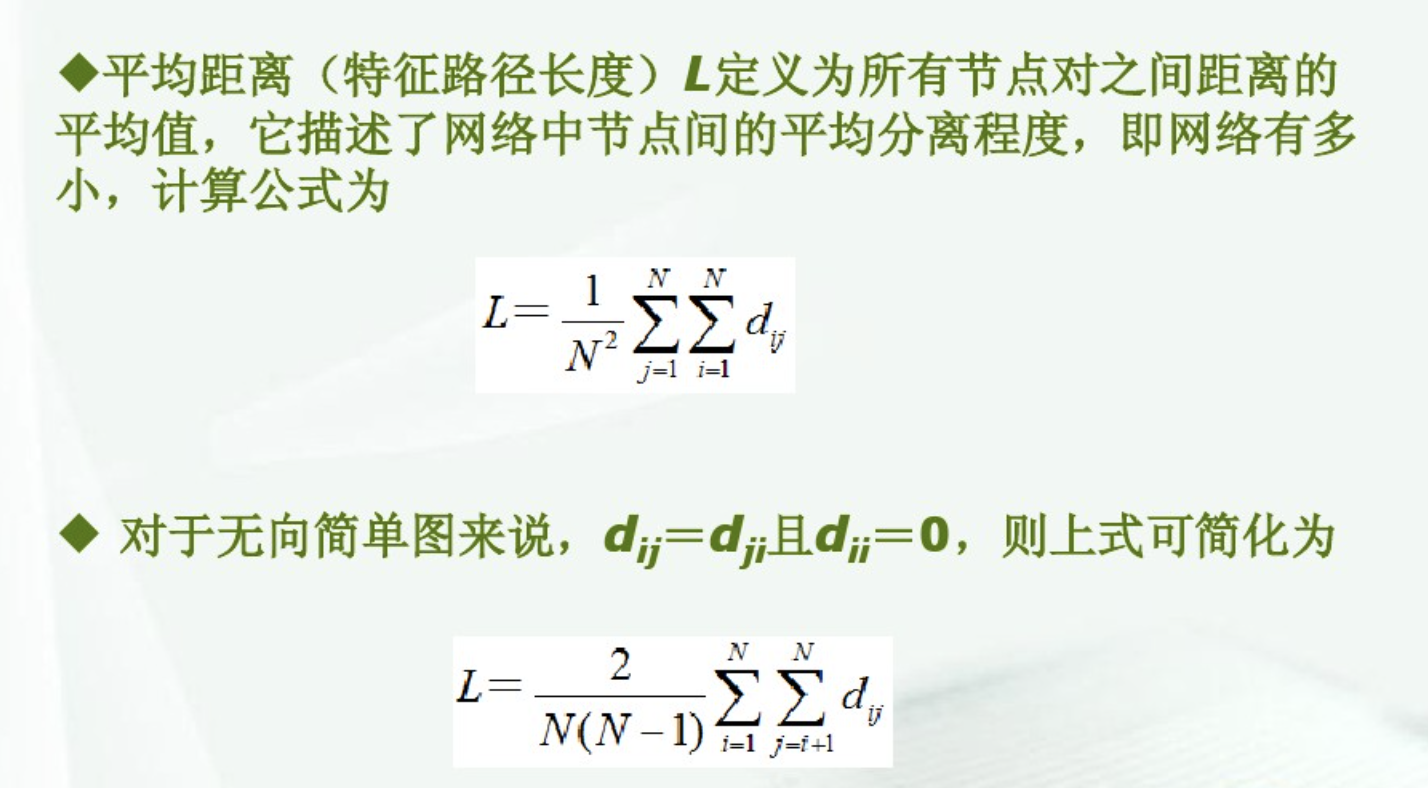• 节点度：无向图中，与节点v 相连的节点数量d(v)或 ${k}_{i}$$k_i$
有向图中 分 出度和入度，入度 其他节点指向节点v的个数 ${d}_{i}n\left(v\right)$$d _ in (v)$
出度，节点v指向其他节点的个数

网络平均度${D}^{-}=av{g}_{i,j}{d}_{\left(}ij\right)$$D^-=avg_{i,j}{d_(ij)}$

度分布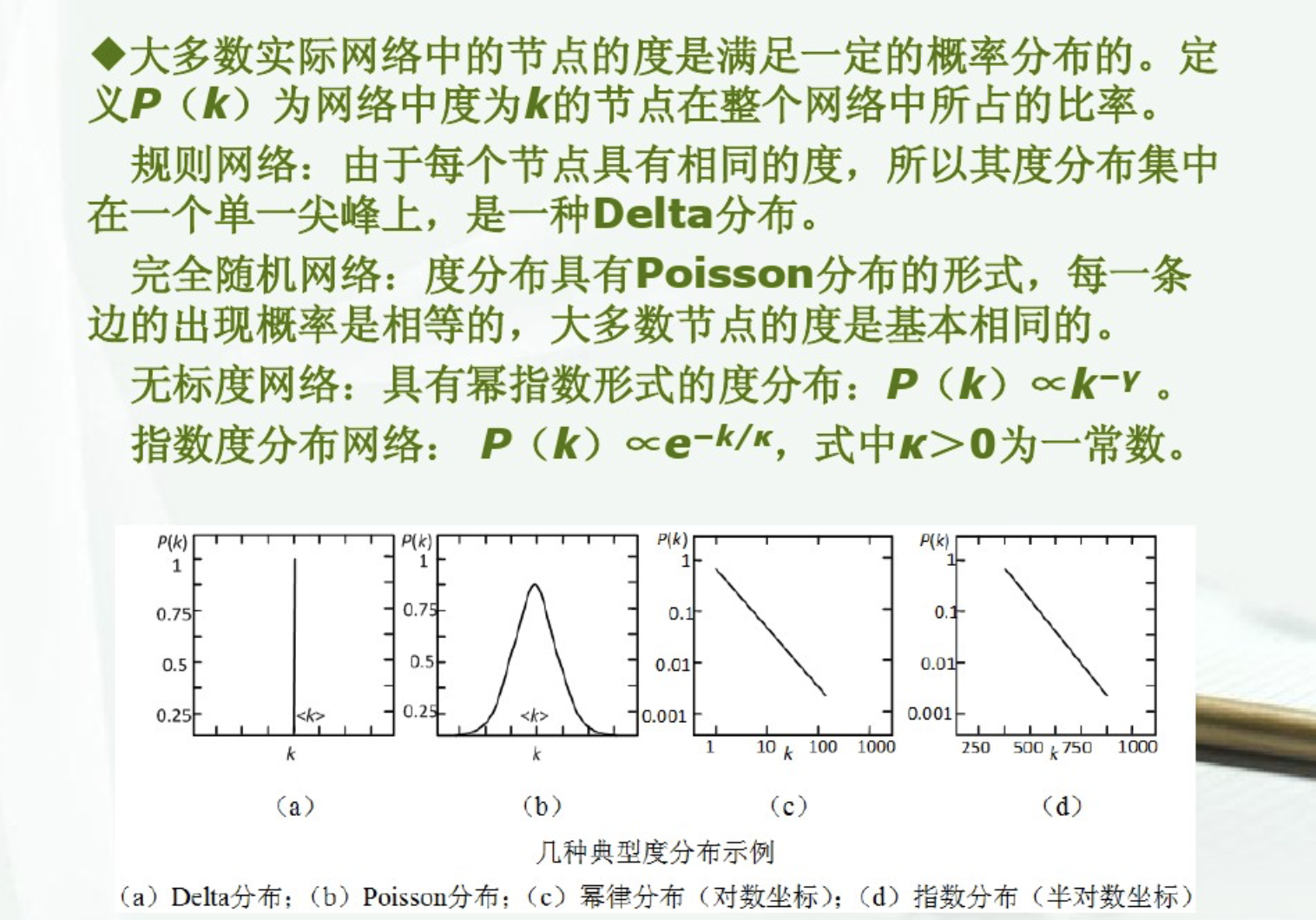累计度分布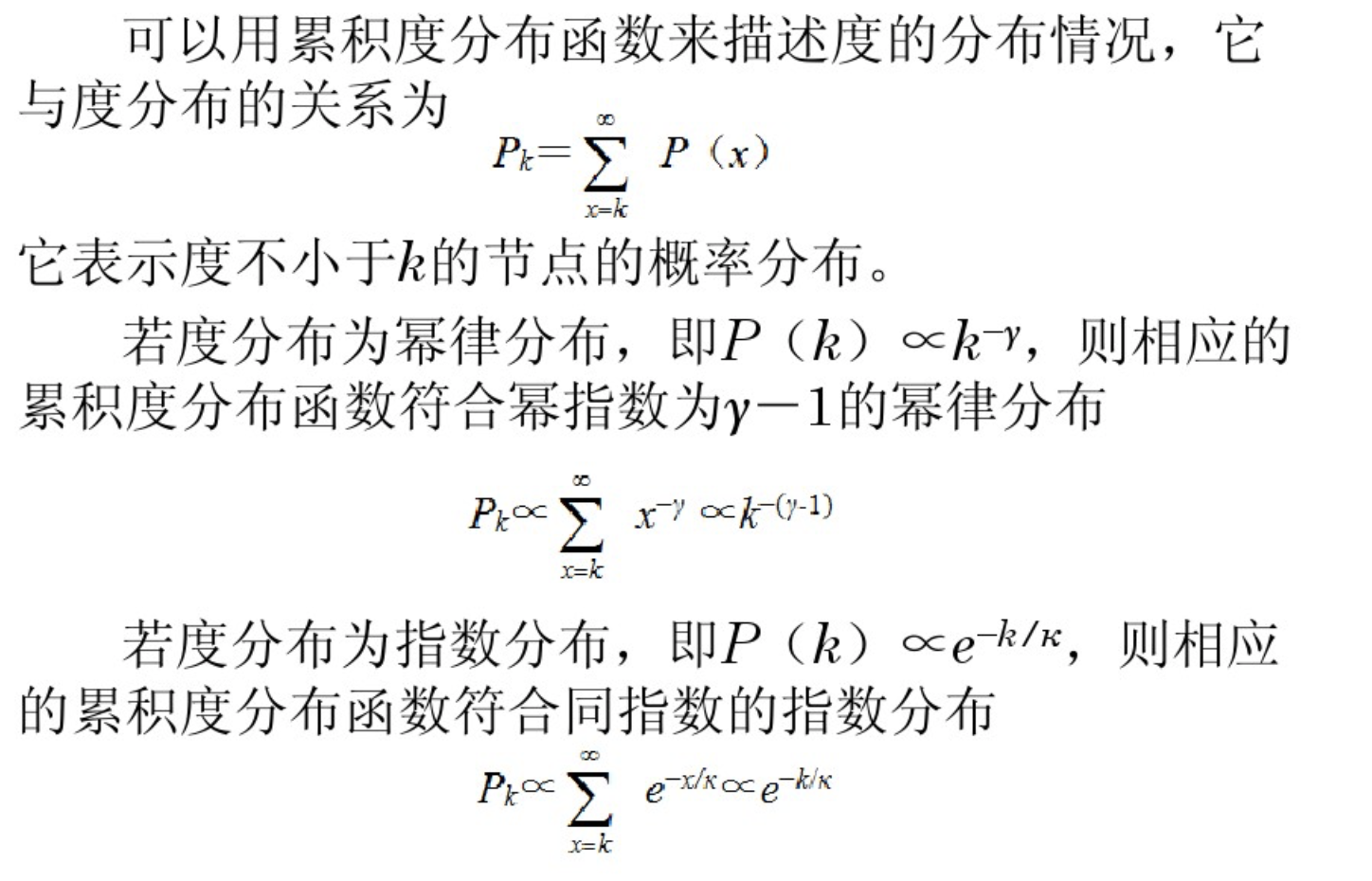• 聚集系数
网络G =（V，E）
d(${v}_{i}$$v_i$)表示${v}_{i}$$v_i$的度值
与节点${v}_{i}$$v_i$相连的顶点有d(${v}_{i}$$v_i$), 这些节点实际相恋的次数为${M}_{i}$$M_i$
最多可能的边数为 $d\left({v}_{i}\right)\left(d\left({v}_{i}\right)-1\right)/2$$d(v_i)(d(v_i)-1)/2$
节点${v}_{i}$$v_i$的聚集系数为
${c}_{i}=\frac{2{M}_{i}}{d\left({v}_{i}\right)\left(d\left({v}_{i}\right)-1\right)}$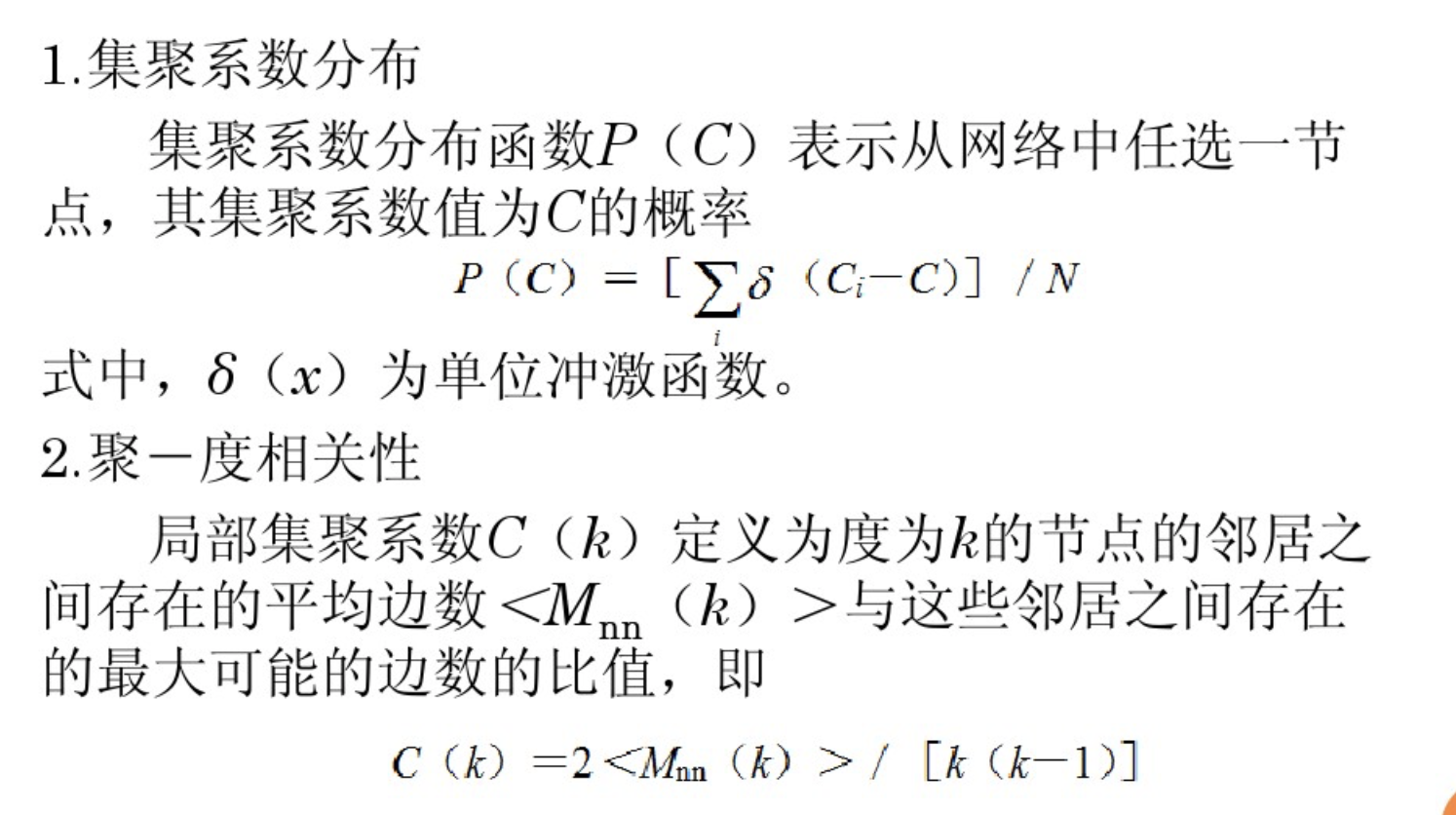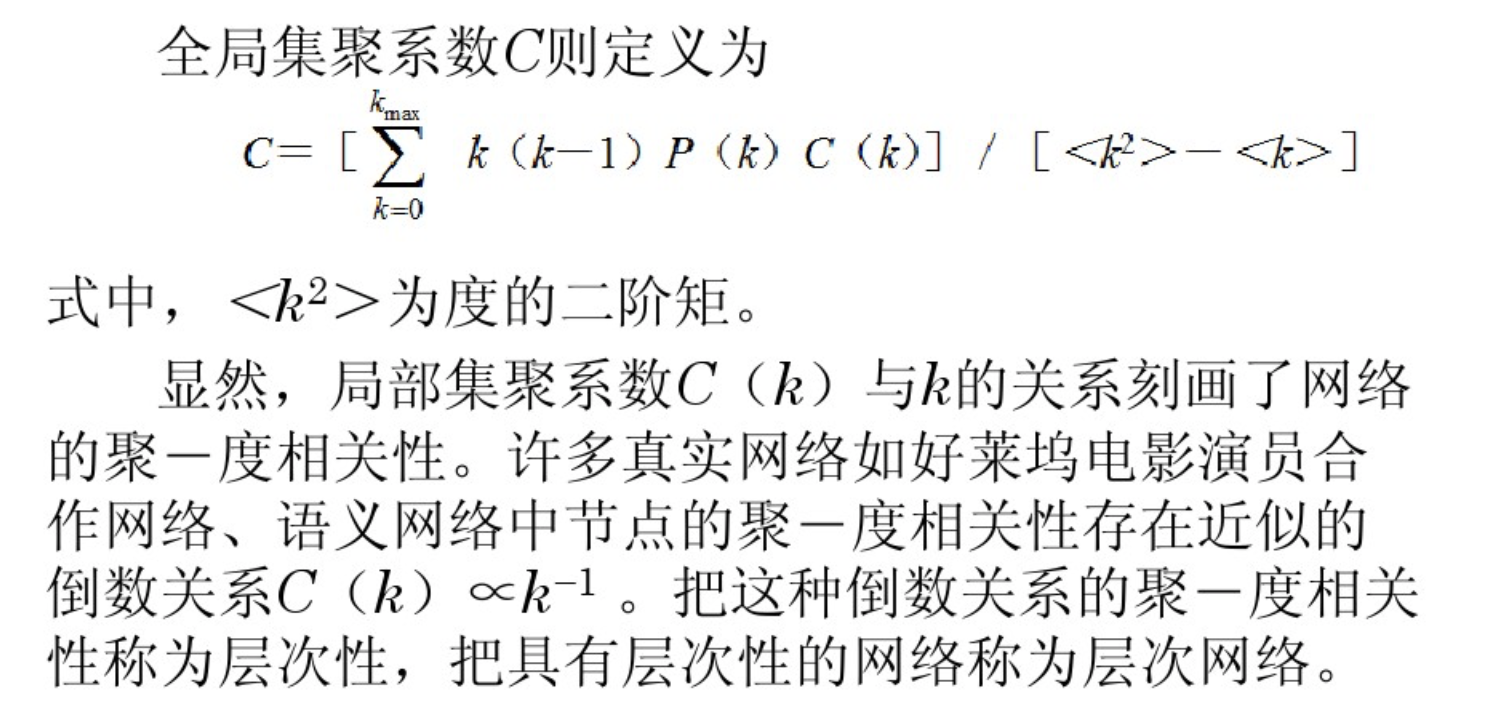• 介数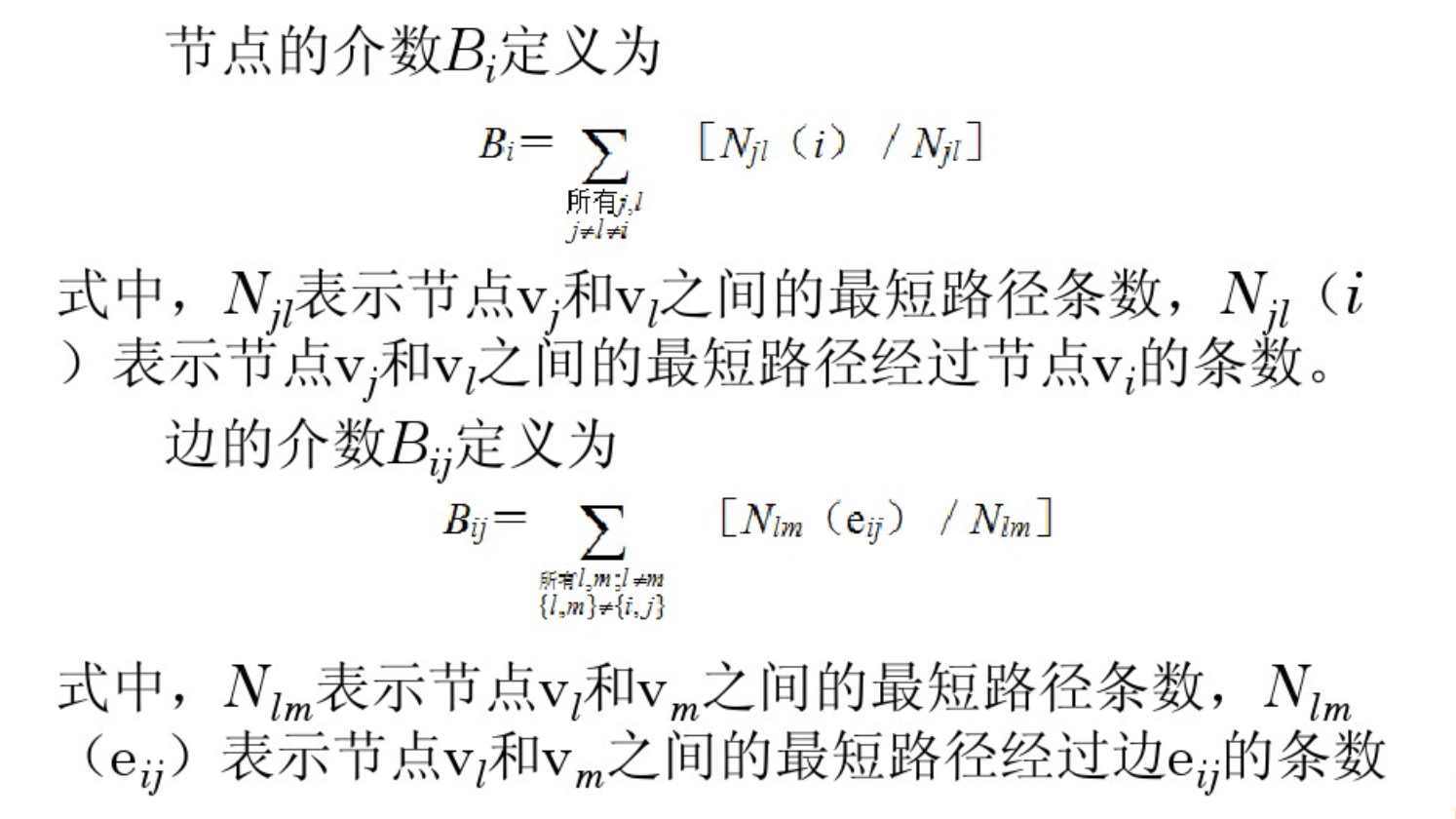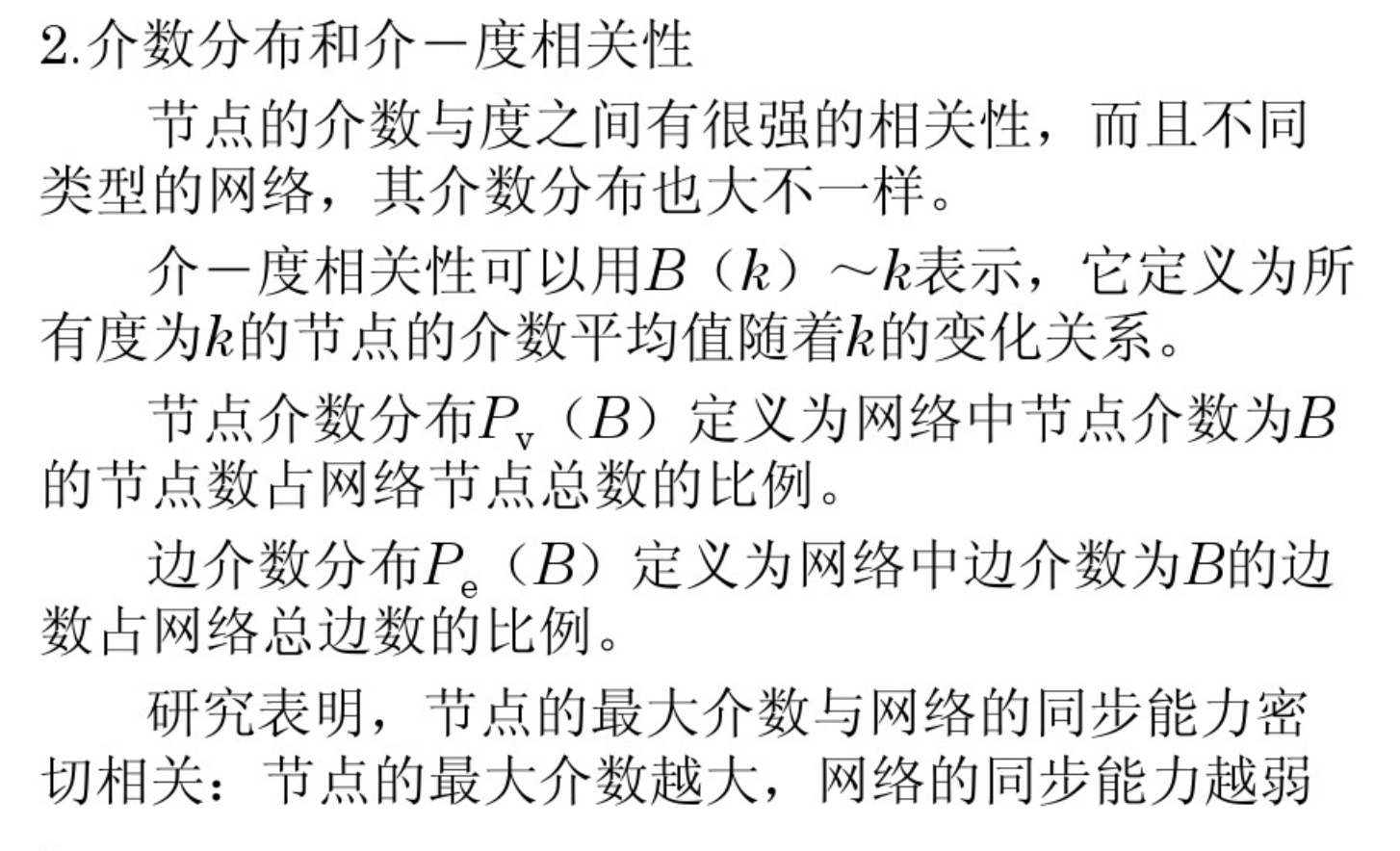• 核数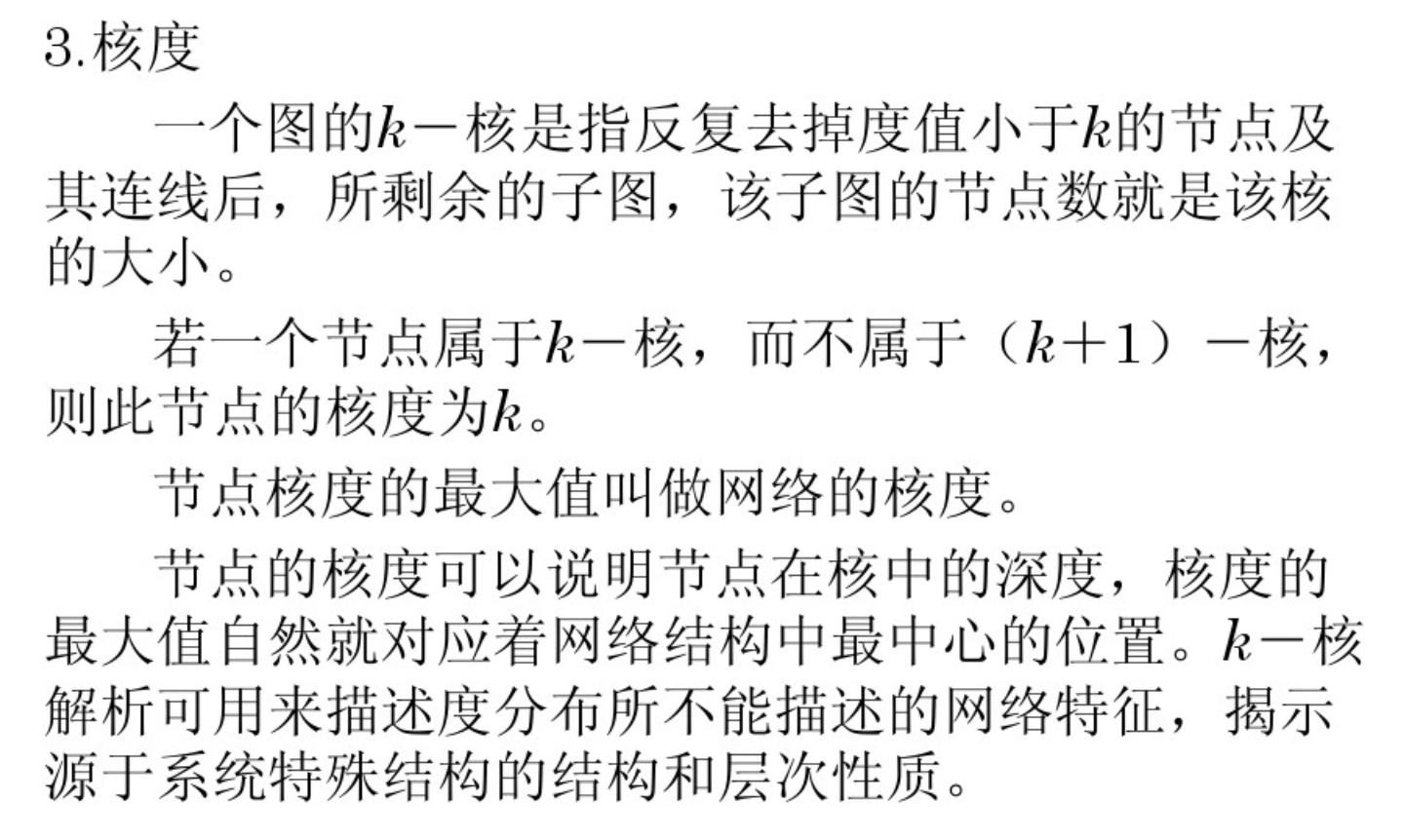• 度中心性
节点${v}_{i}$$v_i$的度中心性：
$d\left({v}_{i}\right)/\left(N-1\right)$

${C}_{D}=\sum _{I=1}^{N}\left[{C}_{D}\left({V}_{m}ax\right)-{C}_{D}\left({V}_{i}\right)\right]/H$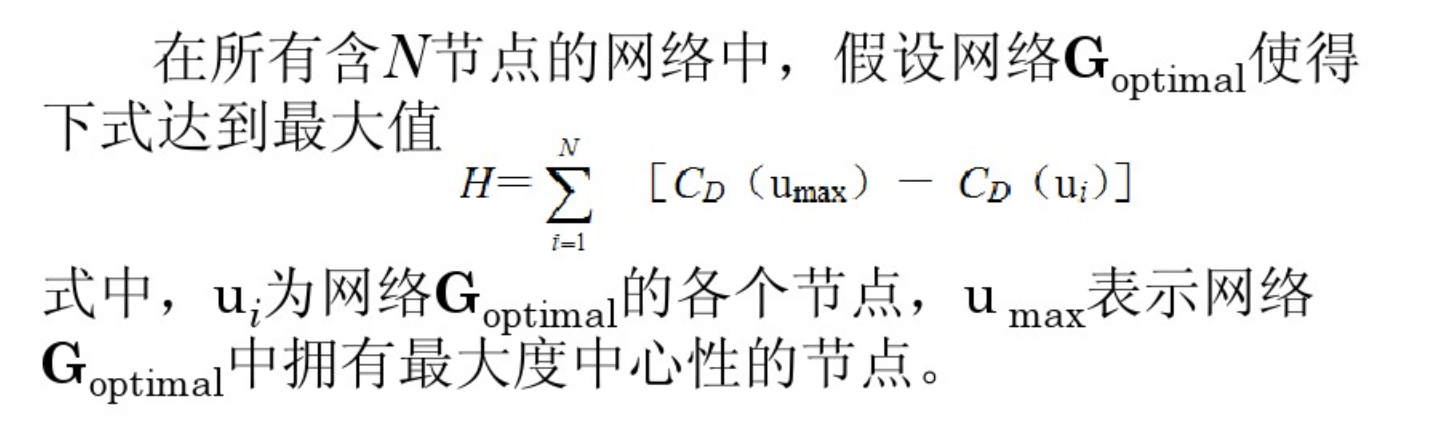• 介数中心性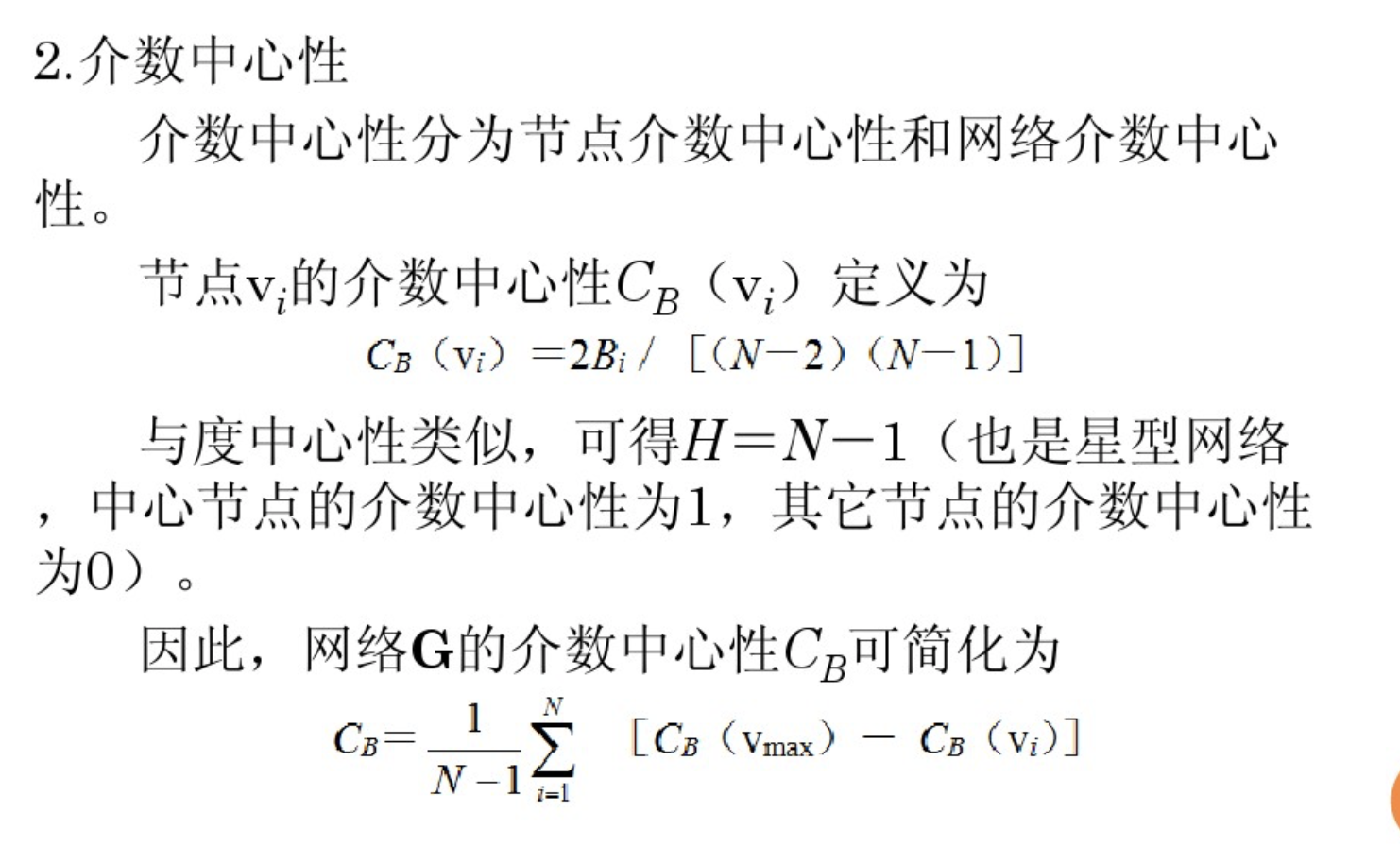• 接近度指标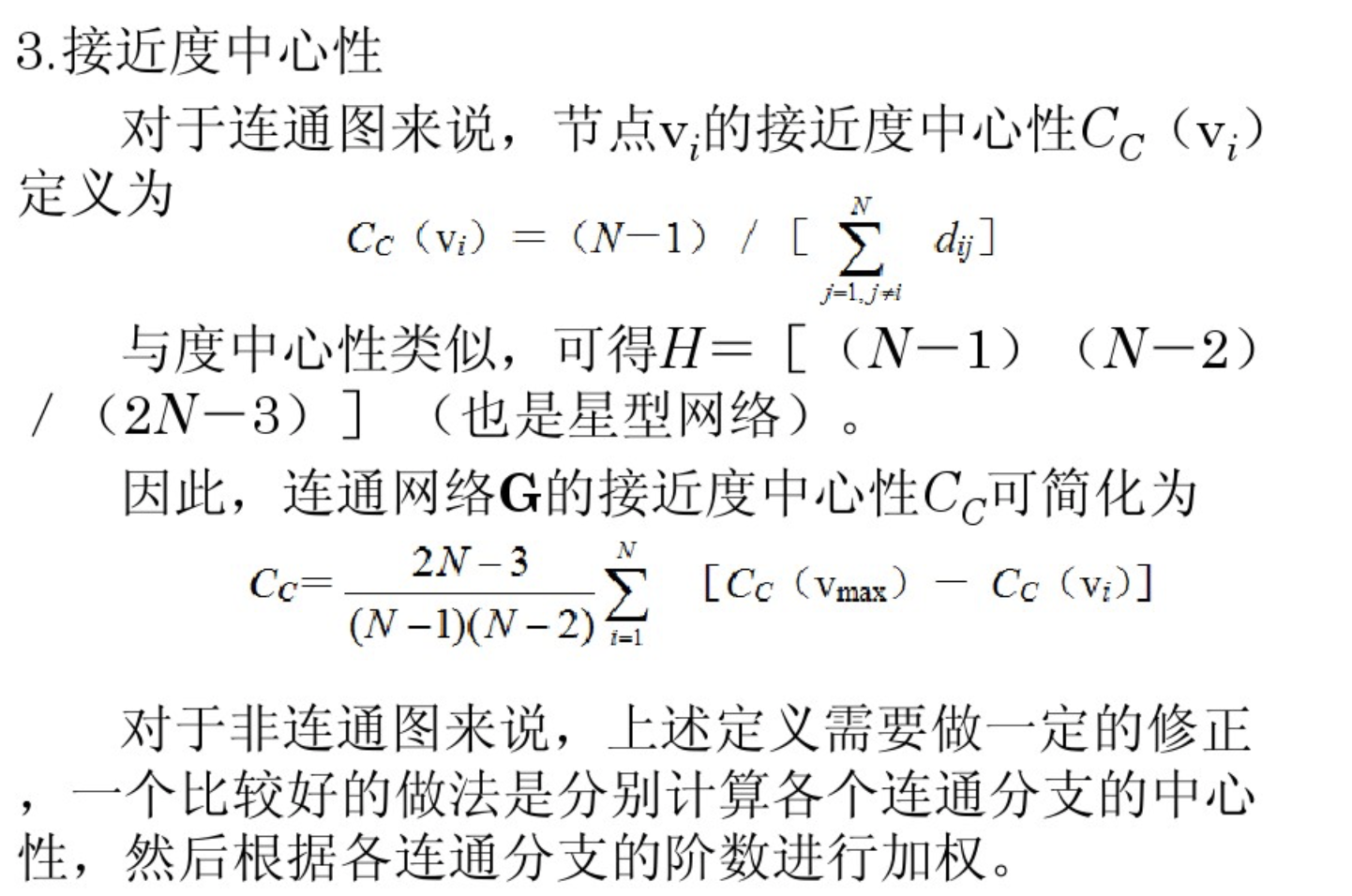• 密度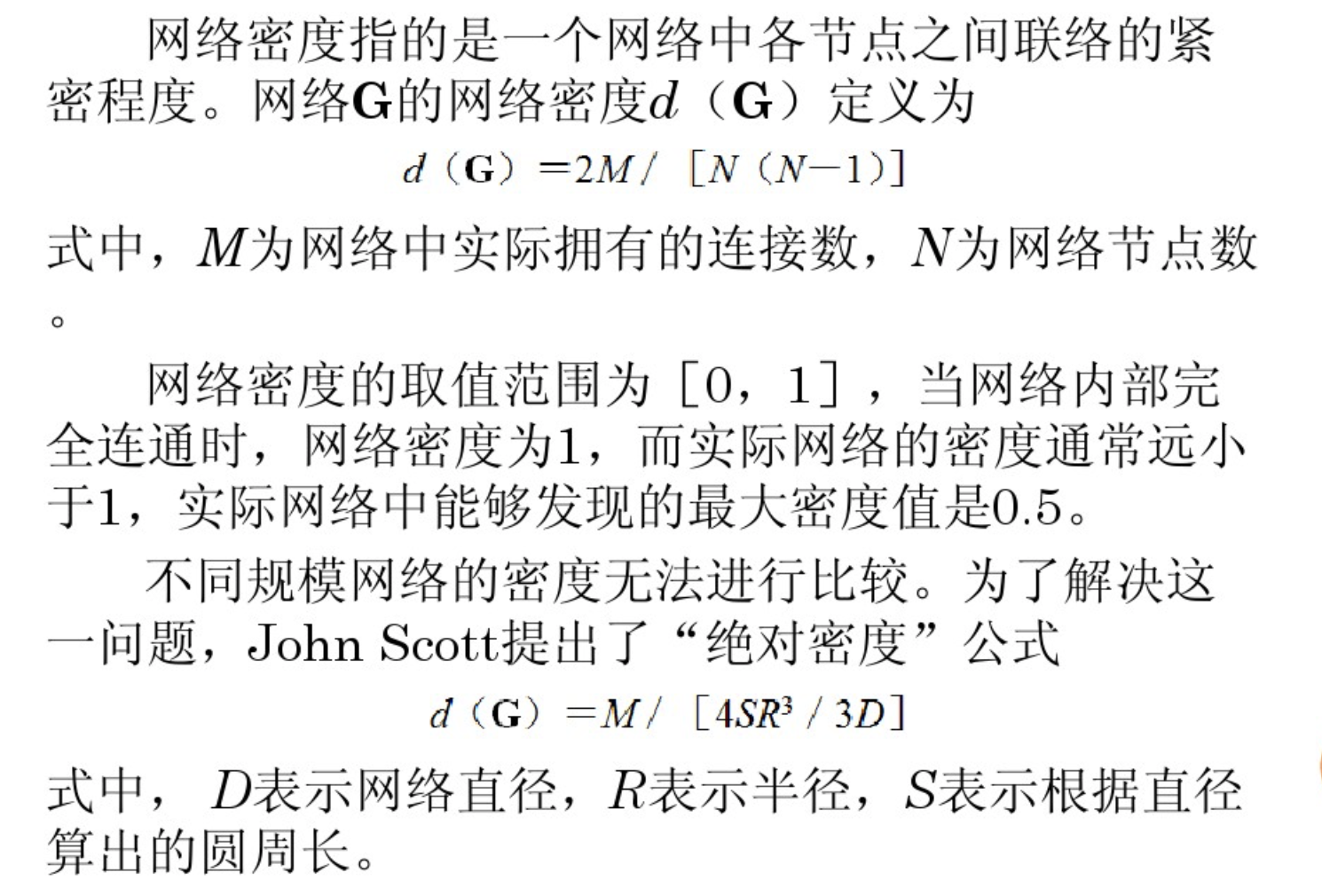## networkx实现

import networkx as nx #使用库的声明
G=nx.Graph()  #初始化
G=nx.Graph(name='my graph')
e=[(1,2),(1,3),(2,3),(3,4),(4,5)]
G=nx.Graph(e)  #为图G添加边  
#节点数
len(G)
##边数
G.number_of_edges()
###节点表
G.nodes()
##边表
G.edges()
Out: EdgeView([(1, 2), (1, 3), (2, 3), (3, 4), (4, 5)])

nx.draw(G)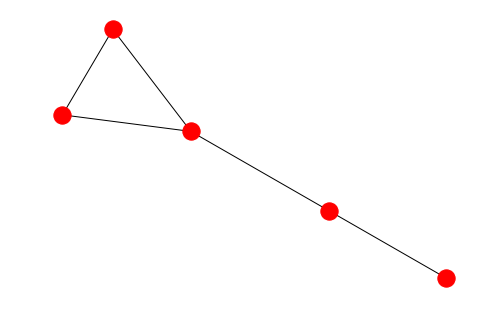diameter(G)
Out: 0.5

shortest_path(G, source=None, target=None, weight=None)

Parameters :

G : NetworkX graph

source : node, optional

Starting node for path. If not specified, compute shortest paths using all nodes as source nodes.

target : node, optional

Ending node for path. If not specified, compute shortest paths using all nodes as target nodes.

weight : None or string, optional (default = None)

If None, every edge has weight/distance/cost 1. If a string, use this edge attribute as the edge weight. Any edge attribute not present defaults to 1.

Returns :
path: list or dictionary
print(nx.shortest_path(G,source=5,target=1))
[5, 4, 3, 1]
p=nx.shortest_path(G,source=1)
p
Out: [1, 2]
#所有节点间的最短*路径*,列表存储
nx.all_pairs_shortest_path(G)
Out: <generator object all_pairs_shortest_path at 0x151ef99c50>
>>> G=nx.path_graph(5)
>>> path=nx.all_pairs_shortest_path(G)
>>> print(path)
[0, 1, 2, 3, 4]
#网络节点间的平均最短路长度
nx.average_shortest_path_length(G)
Out: 1.7

Degree_distribution = nx.degree_histogram(G)
A list of frequencies of degrees. The degree values are the index in the list.
Out: [0, 1, 3, 1]

$d\left({v}_{i}\right)/\left(N-1\right)$

nx.degree_centrality(G)
{1: 0.5, 2: 0.5, 3: 0.75, 4: 0.5, 5: 0.25}  

#插入边，点会自动生成
G.add_edge(6,7 )

${c}_{i}=\frac{2{M}_{i}}{d\left({v}_{i}\right)\left(d\left({v}_{i}\right)-1\right)}$

nx.clustering(G)
{1: 1.0, 2: 1.0, 3: 0.3333333333333333, 4: 0, 5: 0, 6: 0, 7: 0}

closing

nx.closeness_centrality(G)
Out:
{1: 0.42857142857142855,
2: 0.42857142857142855,
3: 0.6,
4: 0.6666666666666666,
5: 0.42857142857142855,
6: 0.5,
7: 0.35294117647058826}

nx.betweenness_centrality(G)

nx.betweenness_centrality(G)
{1: 0.0,
2: 0.0,
3: 0.5333333333333333,
4: 0.7333333333333333,
5: 0.0,
6: 0.3333333333333333,
7: 0.0}

nx.transitivity(G)

0.3333333333333333

## 绘图

"""
Created on Thu Jul 19 20:49:23 2018

@author: cc
"""

import networkx as nx                   #导入networkx包
import matplotlib.pyplot as plt     #导入绘图包matplotlib（需要安装，方法见第一篇笔记）
G =nx.random_graphs.barabasi_albert_graph(100,1)   #生成一个BA无标度网络G
nx.draw(G)                          #绘制网络G
plt.savefig("ba.png")           #输出方式1: 将图像存为一个png格式的图片文件
plt.show()                            #输出方式2: 在窗口中显示这幅图像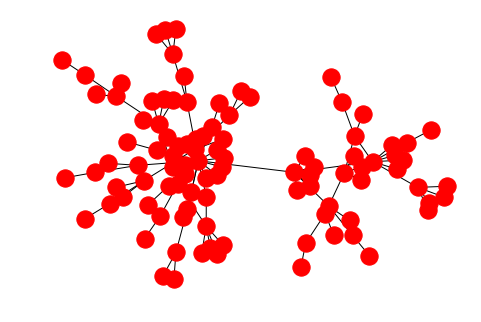- node_size: 指定节点的尺寸大小(默认是300，单位未知，就是上图中那么大的点)
- node_color: 指定节点的颜色 (默认是红色，可以用字符串简单标识颜色，例如’r’为红色，’b’为绿色等，具体可查看手册)
- node_shape: 节点的形状（默认是圆形，用字符串’o’标识，具体可查看手册）
- alpha: 透明度 (默认是1.0，不透明，0为完全透明)
- width: 边的宽度 (默认为1.0)
- edge_color: 边的颜色(默认为黑色)
- style: 边的样式(默认为实现，可选： solid|dashed|dotted,dashdot)
- with_labels: 节点是否带标签（默认为True）
- font_size: 节点标签字体大小 (默认为12)
- font_color: 节点标签字体颜色（默认为黑色）

NetworkX在绘制网络图形方面提供了布局的功能，可以指定节点排列的形式。这些布局包括：
circular_layout：节点在一个圆环上均匀分布
random_layout：节点随机分布
shell_layout：节点在同心圆上分布
spring_layout： 用Fruchterman-Reingold算法排列节点（这个算法我不了解，样子类似多中心放射状）
spectral_layout：根据图的拉普拉斯特征向量排列节点？我也不是太明白

http://networkx.lanl.gov/examples/drawing/knuth_miles.html

draw(G, pos=None, ax=None, hold=None, **kwds)
Parameters :
G : graph

A networkx graph

pos : dictionary, optional

A dictionary with nodes as keys and positions as values. If not specified a spring layout positioning will be computed. See networkx.layout for functions that compute node positions.

ax : Matplotlib Axes object, optional

Draw the graph in specified Matplotlib axes.

hold : bool, optional

Set the Matplotlib hold state. If True subsequent draw commands will be added to the current axes.

**kwds : optional keywords

See networkx.draw_networkx() for a description of optional keywords.

## 参考文献

https://networkx.github.io/documentation/networkx-1.9/reference/generated/networkx.classes.function.degree_histogram.html?highlight=degree_histogram

https://www.cnblogs.com/gispathfinder/p/5790949.html